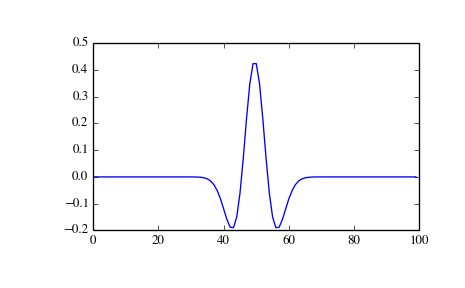# scipy.signal.ricker¶

scipy.signal.ricker(points, a)[source]

Return a Ricker wavelet, also known as the “Mexican hat wavelet”.

It models the function:

A (1 - x^2/a^2) exp(-x^2/2 a^2),

where A = 2/sqrt(3a)pi^1/4.

Parameters: points : int Number of points in vector. Will be centered around 0. a : scalar Width parameter of the wavelet. vector : (N,) ndarray Array of length points in shape of ricker curve.

Examples

```>>> from scipy import signal
>>> import matplotlib.pyplot as plt
```
```>>> points = 100
>>> a = 4.0
>>> vec2 = signal.ricker(points, a)
>>> print(len(vec2))
100
>>> plt.plot(vec2)
>>> plt.show()
```scipy.signal.qmf

scipy.signal.cwt